# How to roll half a dice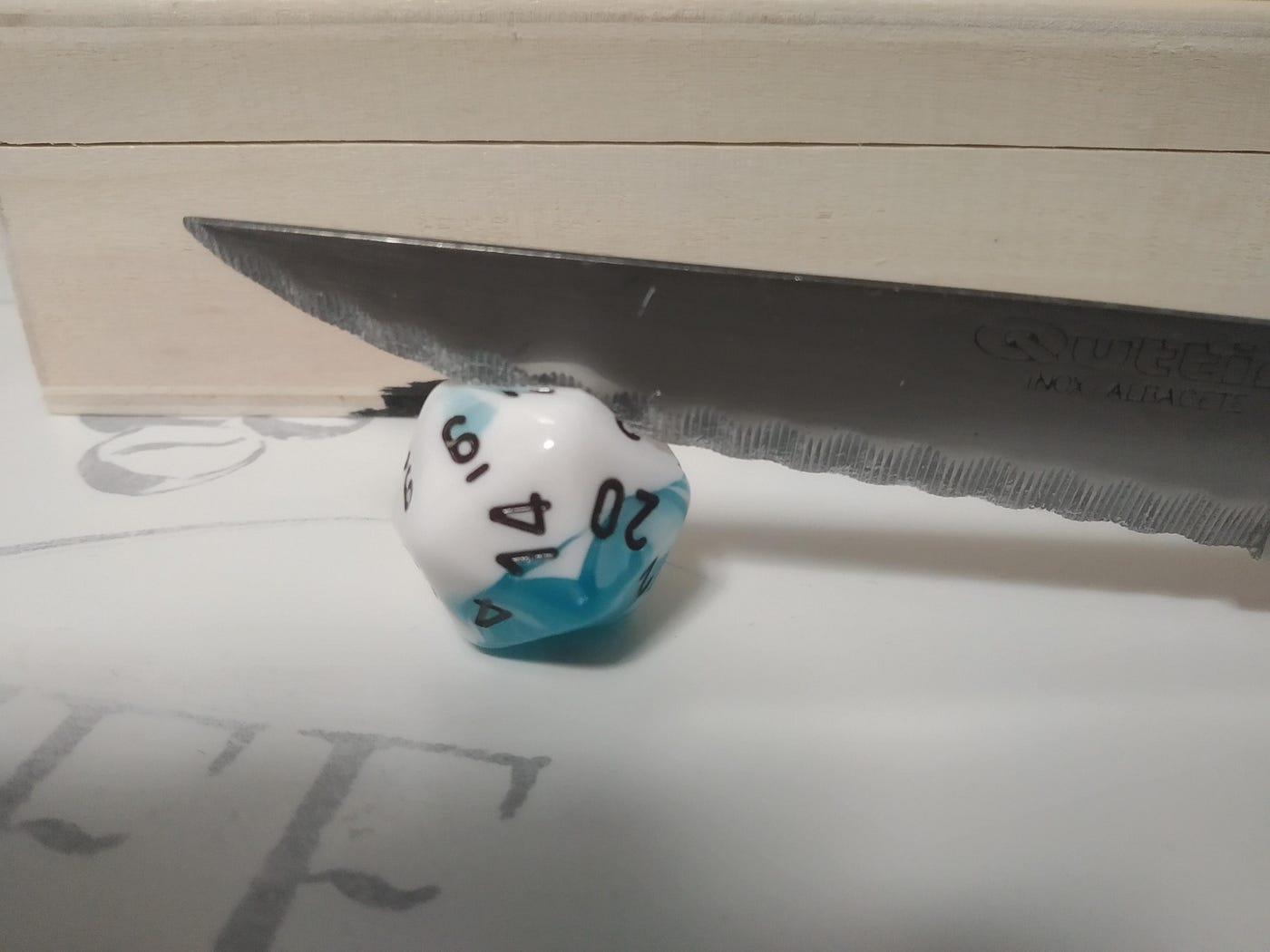via reddit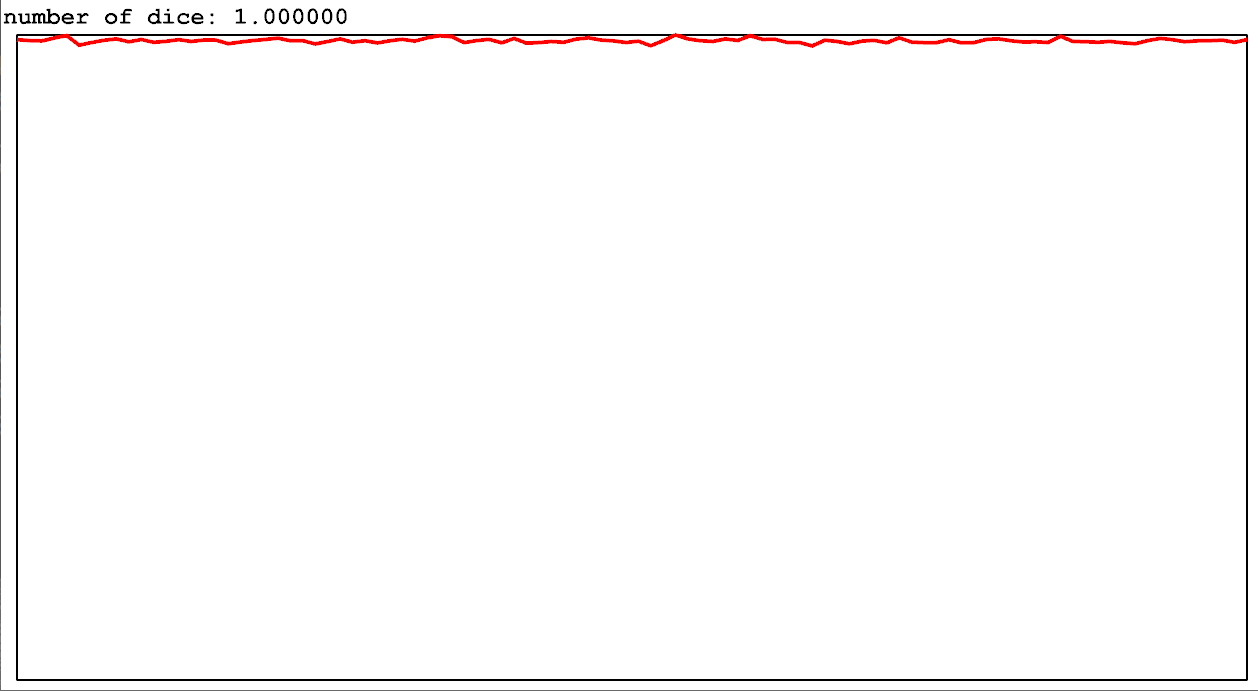function: roll N continuous dice, take the max result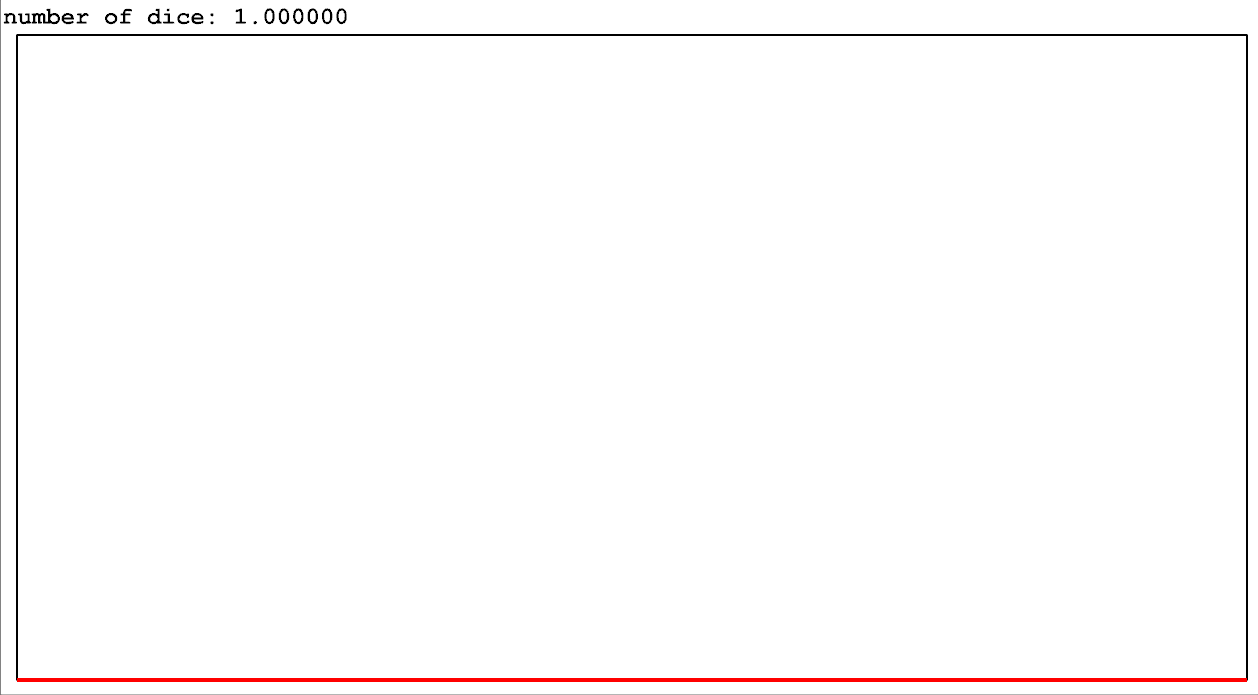function: roll one continuous dice, apply f(x) = x^(n-1) to it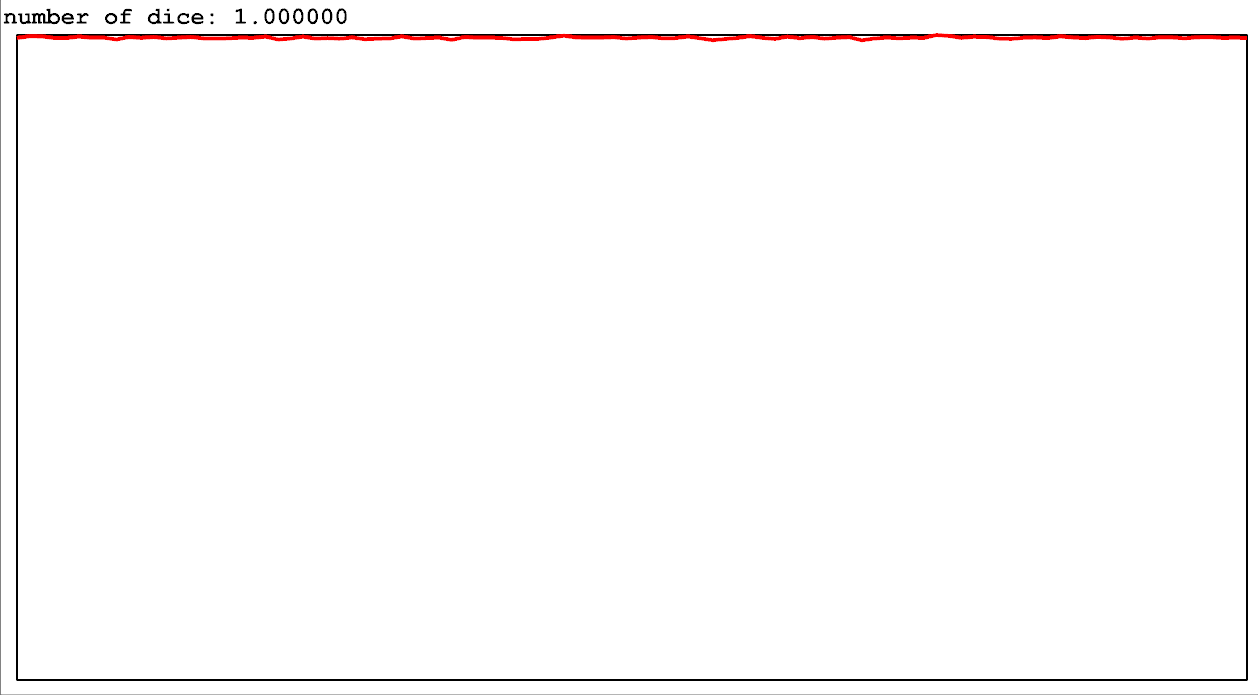function: roll one continuous dice, apply f(x) = x^(1/n) to it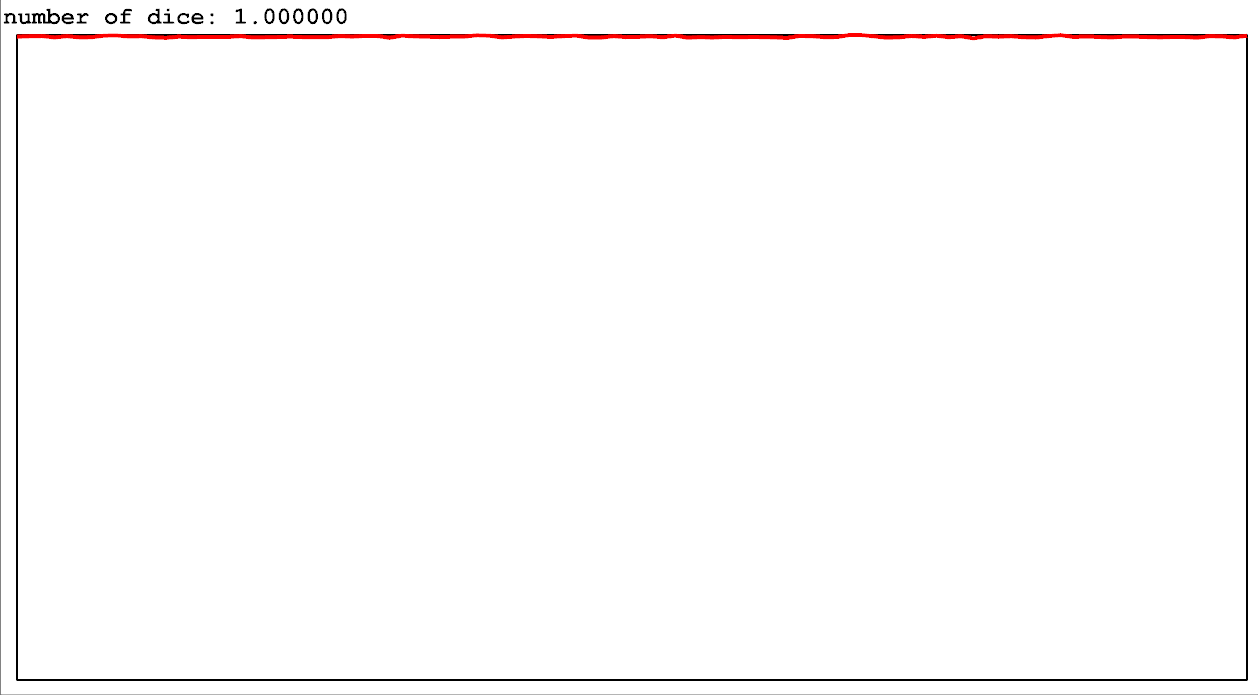function: roll one continuous dice, apply f(x) = x^(1/n) to it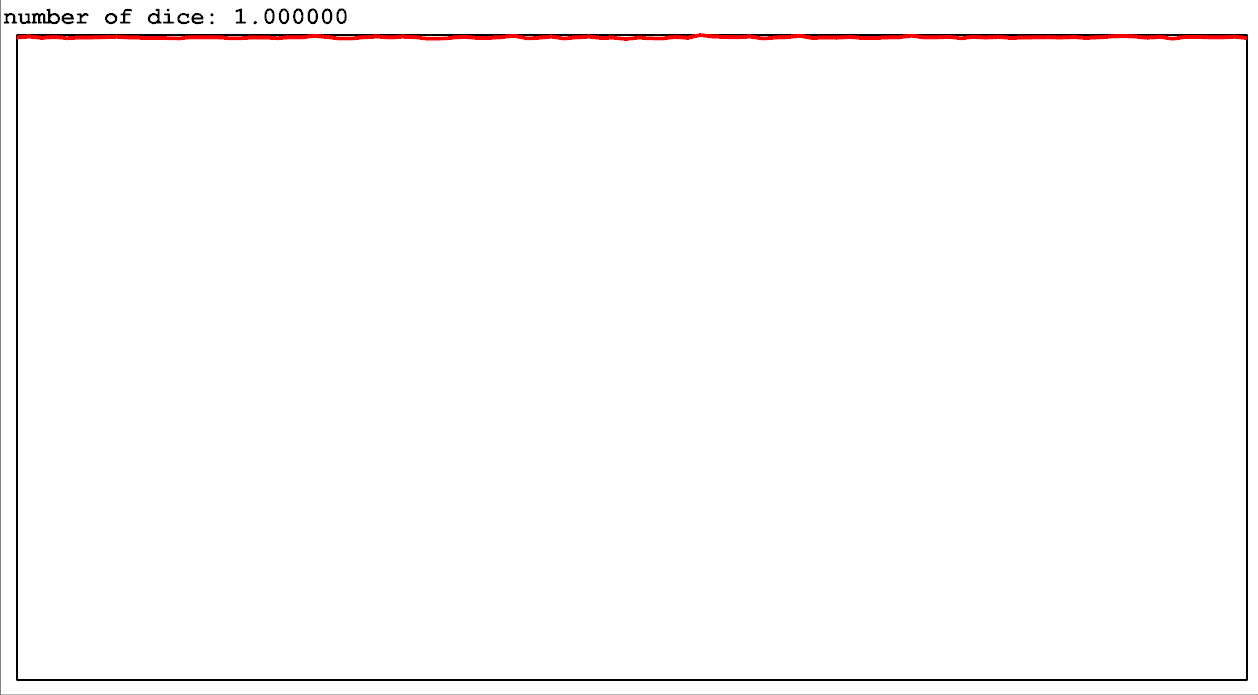function: take p = the fractional portion of n. 1-p chance of floor(n) dice rolls, p chance of ceil(n) dice rolls

--

--